×#### Thank you for registering.

One of our academic counsellors will contact you within 1 working day.

Click to Chat

1800-1023-196

+91-120-4616500

CART 0

• 0

MY CART (5)

Use Coupon: CART20 and get 20% off on all online Study Material

ITEM
DETAILS
MRP
DISCOUNT
FINAL PRICE
Total Price: Rs.

There are no items in this cart.
Continue Shopping• Kinematics & Rotational Motion
• OFFERED PRICE: Rs. 636
• View Details

```Dimensional Analysis and its Applications

Table of Content

Introduction to Dimensional Analysis and its Applications

Dimensional Analysis

Principle of homogeneity

Applications of Dimensional Analysis

Consistency of a Dimensional Equation

Derive relations between physical quantities involved in physical phenomena

To change units from one system to another

Introduction to Dimensional Analysis and its Applications

Understanding dimensions is of utmost importance as it helps us in studying the nature of physical quantities mathematically. The basic concept of dimensions is that we can add or subtract only those quantities which have same dimensions. Also, two physical quantities are equal if they have same dimensions. These basic ideas help us in deriving the new relation between physical quantities, it is just like units.

Dimensional Analysis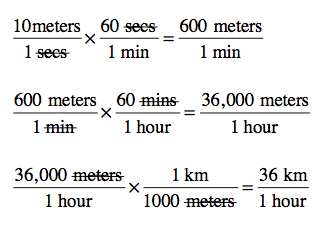Image 1: Use of conversion factors

The study of relationships between physical quantities with the help of their dimensions and units of measurements is called dimensional analysis. We use dimensional analysis in order to convert a unit from one form to another. In science and mathematical problems, we have to keep the unit same so that we can perform the mathematical operations easily.

Dimensional Analysis is also called Factor Label Method or Unit Factor Method as we use conversion factor in order to get same units. As an example, if we want to know how many minutes are in 3 hours, what do we do? We generally think as follows:

1 hour = 60 minutes

3 hour = 3 × 60 minutes = 180 minutes

(here the conversion factor 60 minutes)

We use conversion factors accordingly so that the answer comes in the desired unit and biased results are avoided.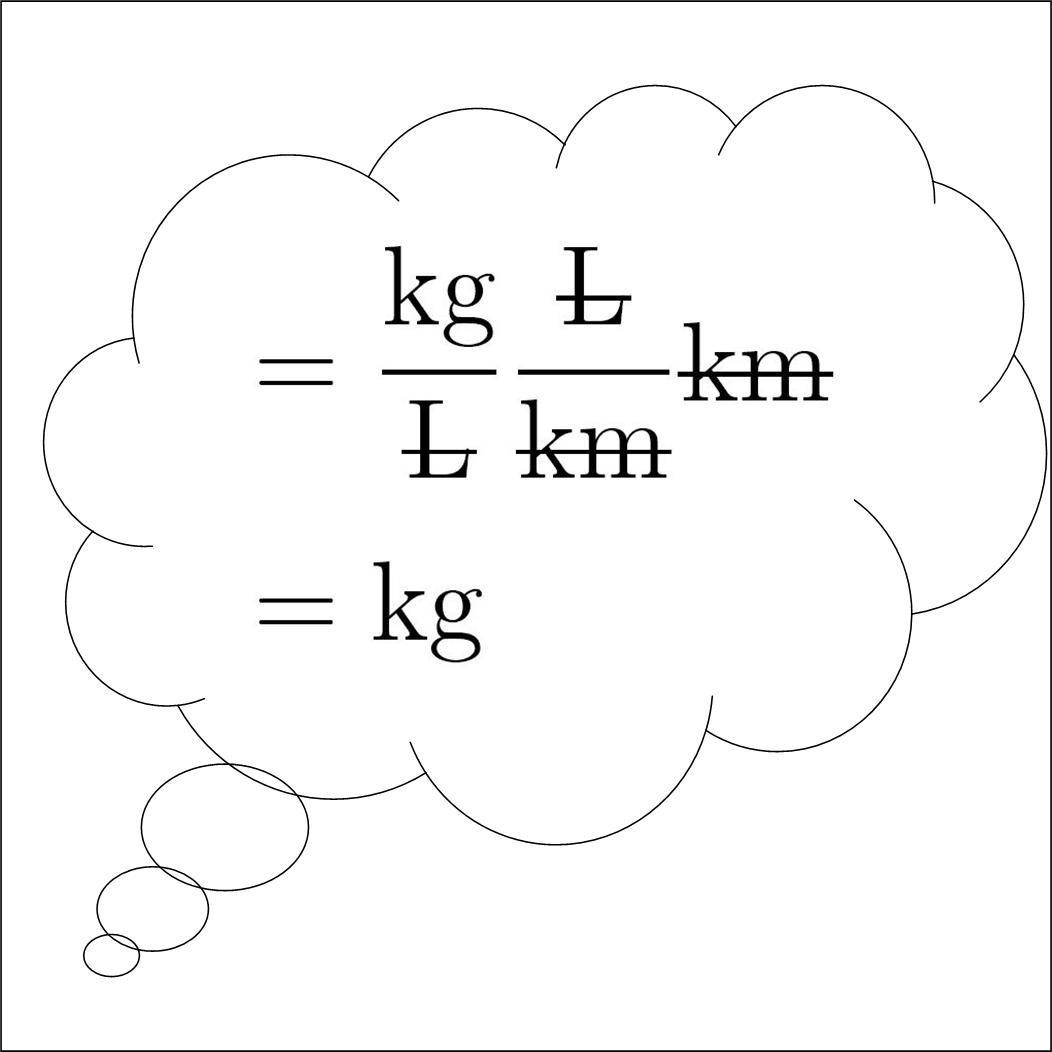Image 2: We only unit which is asked in conversion question, that is, if the answer is asked in kg, it has to be in kg.

Example 1:

To find out how many kilograms are there are 52 pounds (lbs.). In this, we have to convert pounds into kilograms. Generally, 1 kg = 2.2046 lbs., we can use this figure as our conversion factor.

Now 2.2046 lbs. = 1 kg

1 lbs. = 1/2.2046 kg

Then 52 lbs. = 52 × 1/2.2046 kg

= 23.58 kg

Example 2:

To find out how many feet are in 140 centimeters (cm). In this, we have to convert centimeters into feet. Since we can’t convert centimeters directly into feet, so we will convert it into inches, and then convert inches to feet. This involves calculation of two conversion factors.

So 1 inch = 2.54 cm

1 cm = 1/2.54 inches

12 inches = 1 feet

12 × 2.54 cm = 1 feet

30.48 cm = 1 feet

1 cm = 1/30.48 feet

Then , 140 cm = 140 × 1/30.48 feet

= 4.59 feet

Principle of homogeneity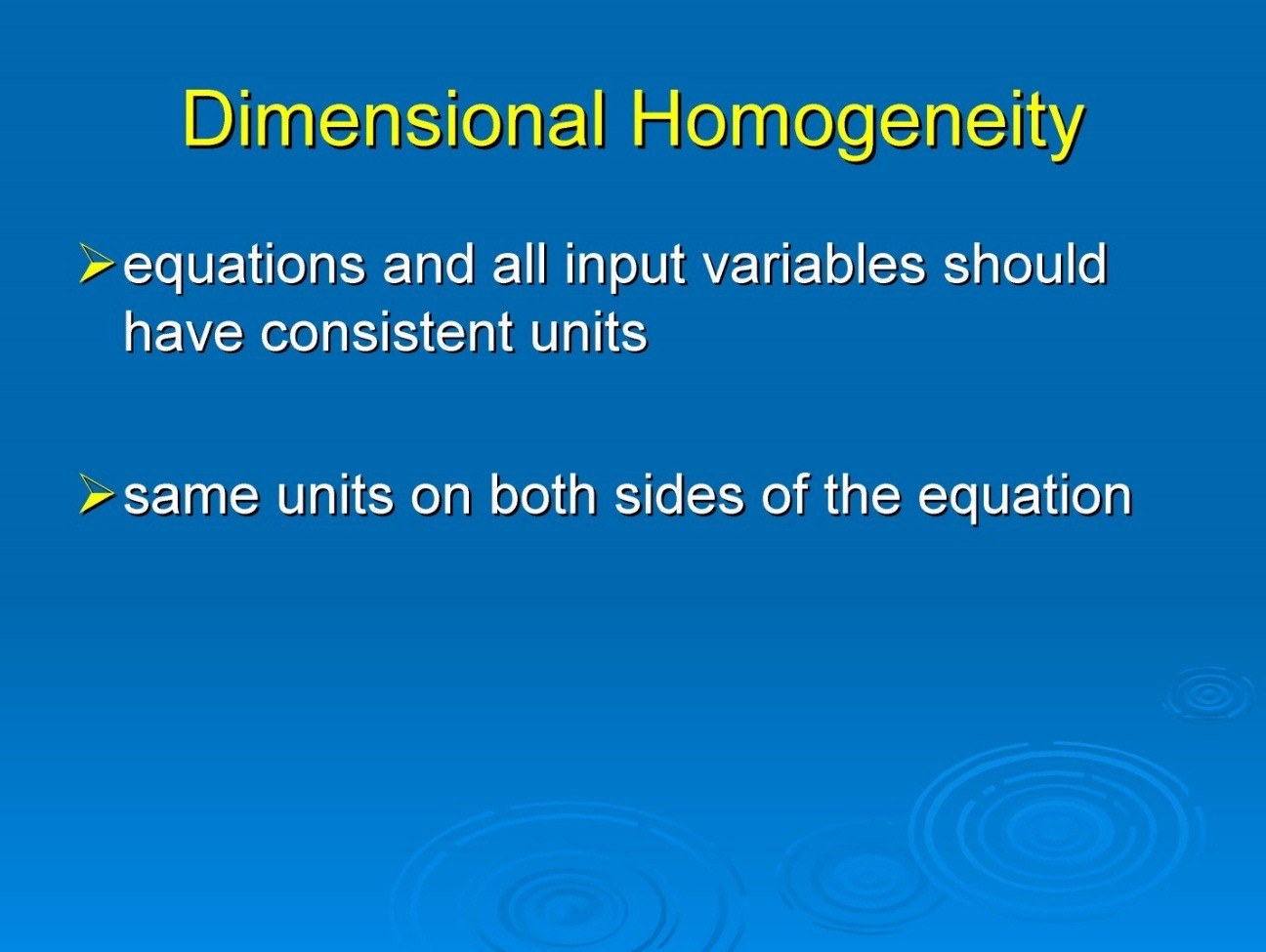Image 3: Concepts of Principle of Homogeneity

The principle of homogeneity states that dimensions of each term of dimension equations are same on both sides.

Applications of Dimensional Analysis

Dimensional Analysis is a very basic aspect of measurement and has many applications in real life physics. We use dimensional analysis for three prominent reasons, they are:

Consistency of a dimensional equation

Derive relation between physical quantities in physical phenomena

To change units from one system to another

Consistency of a Dimensional Equation

We can add or subtract physical quantities only if they have same dimensions. The similarity of dimensions of physical quantities is called consistency of a dimensional equation. As an example take mass and velocity, we can’t add or subtract these two physical quantities as they are of different dimensions. That is [M] for mass and [LT-1] for velocity.

A dimension equation is said to be consistent, only and only if dimensions of equations are same on both sides. If dimensions of the equation are not same on both sides then the equation is said to be dimensionally incorrect. Also, remember that if an equation is dimensionally correct it doesn’t mean it is a completely correct equation.

Example:

Check consistency of dimensional equation of speed.

Speed = Distance/Time

[LT-1] = L/T

[LT-1] = [LT-1]

The equation is dimensionally correct, as the dimension of speed is same on both sides. Dimensional Analysis is a basic test to find out the consistency of equation and doesn’t guarantee the correctness of equation. One drawback of this method is that we can’t predict constants of many physical quantities. Also, the logarithmic, trigonometric and exponential function is dimensionless. For Example, take

Sine of angle = Length/Length is unitless; therefore it is a dimensionless quantity.

Example 1

Check the consistency of the equation

x = x0 + v0t + (1/2) at2

where x and x0 are distances, t is time, v is velocity and a is an acceleration of the body.

Now to check if the above equation is dimensionally correct, we have to prove that dimensions of physical quantities are same on both sides. Also, we have to keep in mind that quantities can only be added or subtracted if their dimensions are same.

x = distance = [L]

x0= distance = [L]

v0t = velocity × time = [LT-1] × [T] = [L]

at2 = acceleration × time2 = [LT-2] × [T2] = [L]

Since dimensions of left hand side equals to dimension on right hand side, equation is said to be consistent and dimensionally correct.

Example 2

Check whether the given equation is dimensionally correct.

W = 1/2 mv2 – mgh

where W stands for work done, m means mass, g stands for gravity, v for velocity and h for height.

To check the above equation as dimensionally correct, we first write dimensions of all the physical quantities mentioned in the equation.

W = Work done = Force × Displacement = [MLT-2] × [L] = [ML2T-2]

1/2 mv2 = Kinetic Energy = [M] × [L2T-2] = [ML2T-2]

mgh = Potential Energy = [M] × [LT-2] × [L] = [ML2T-2]

Since all the dimensions on left and right sides are equal it is a dimensionally correct equation.

Derive relations between physical quantities involved in physical phenomena

The method of dimension analysis can also help in finding out relations between physical quantities. If we know how physical quantities depend on each other, we can find the relation between them easily by equating dimension on both sides.

Example

Suppose a bob is hanging from a ceiling and time period of oscillations depends on ‘length “l” of the thread, mass “m” of the bob and gravity “g”. In order to find a relation between time and other physical quantities, we proceed as follows:

Let’s time depends on powers x, y and z of length l, mass m and gravity g of the bob. Then the equation becomes

T = k lxmygz where k is a proportionality constant

Writing dimensions on both sides, we get

[M0L0T] = k [L]x[M]y[LT-2]z

Arranging powers accordingly we get

[M0L0T] = k [MyLx+zT-2z]

Equating powers on both sides, we get three equations

y = 0

x + z = 0

-2z = 1

Solving the linear equations we get x = 1/2, y = 0 and z = -1/2

Substituting the values of x, y and z in the equation we have derived the following relation:

T = k L1/2M0g-1/2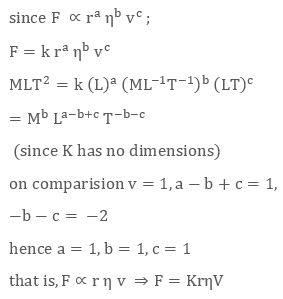Image 4: We form linear equations by equating dimensions on both sides of the equation

To change units from one system to another

Dimensional analysis is also used in obtaining the value of the physical quantity in another system. For Example, if we want to convert a physical quantity from S.I or metric system to C.G.S system we can easily do that with the help of dimensional analysis.

A physical quantity has two parts; one is the numerical or magnitude part and the other part is the unit part. Suppose there’s a physical quantity X, which has unit “U” and magnitude “N”, then it will be expressed as:

X = NU

To convert a physical quantity from one unit to another we use below relation:

N1U1 = N2U2

where N1 and N2 are numerical parts and U1 and U2 are dimensions or units of both quantities.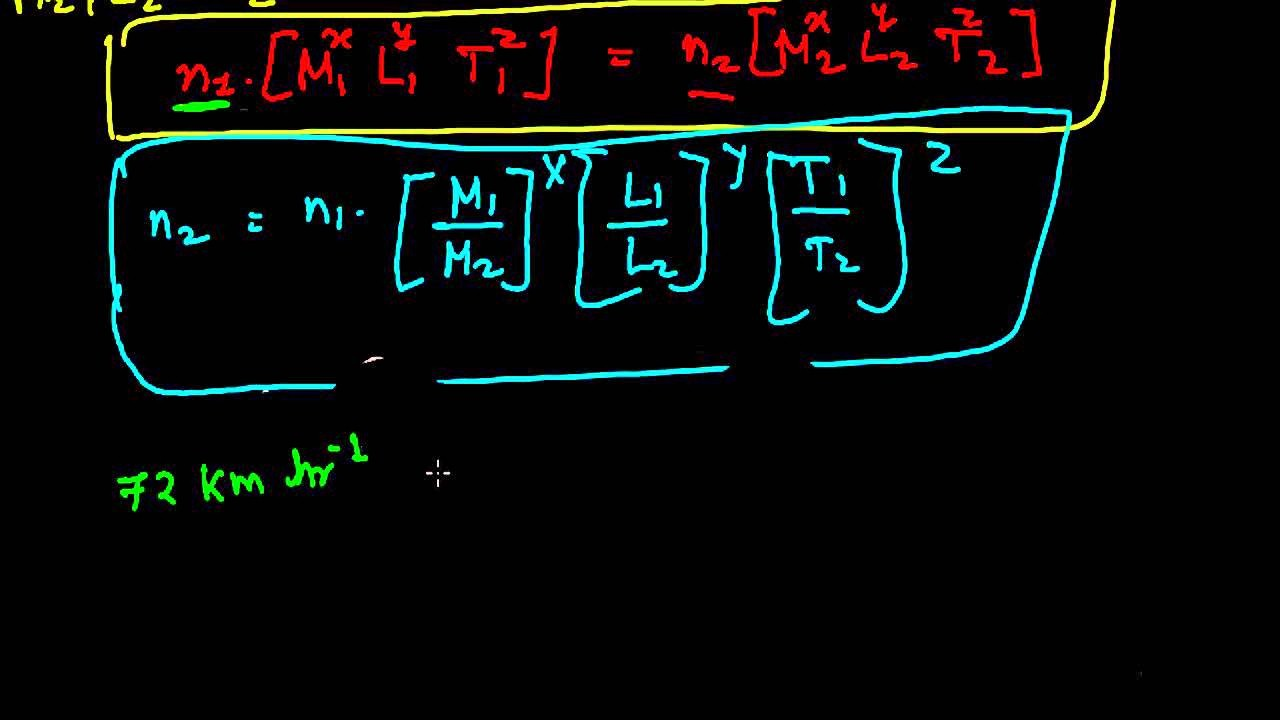Image 5: Use of dimensional analysis in converting units from one system to another.

Example:

Convert force of unit Newton into dyne.

Let N1 and U1 be numerical value and unit of force in S.I system (Newton) and N2 and U2 be numerical value of force in CGS system (Dyne)

N1[MLT-2] = N2[MLT-2]

N1 = 1 [Mass in gm/Mass in kg × Length in cm/Length in m × (Time in seconds/Time in seconds)-2]

N1 = [ gm/1000 gm × cm/100cm × (1 second/ 1 second)-2]

N1 = [ 10-5]

Which means 1 Newton = 10-5 Dyne

Watch this Video for more reference

Dimensional Analysis and its Application
```### Course Features

• 731 Video Lectures
• Revision Notes
• Previous Year Papers
• Mind Map
• Study Planner
• NCERT Solutions
• Discussion Forum
• Test paper with Video Solution